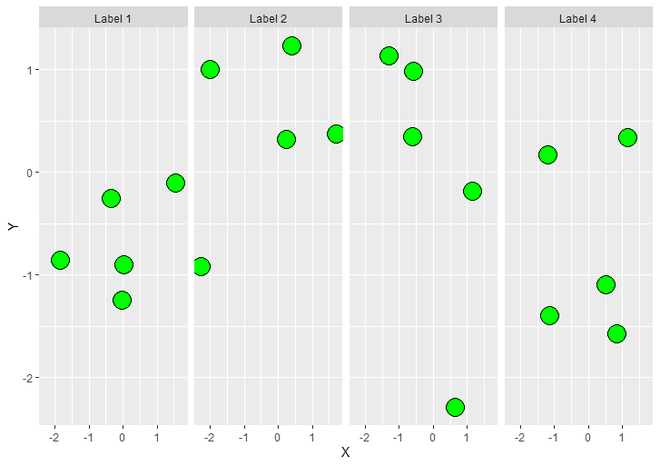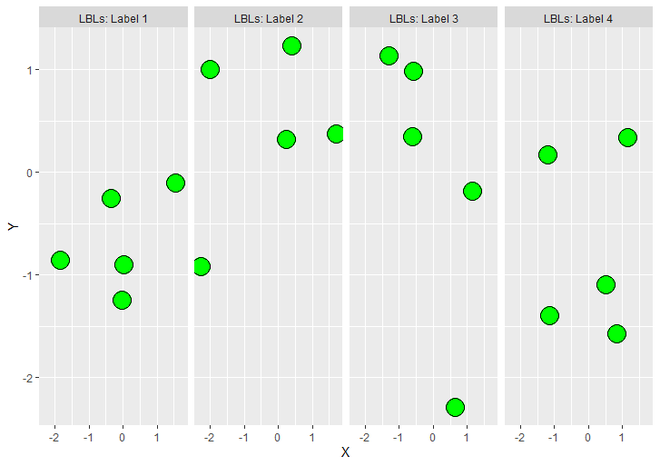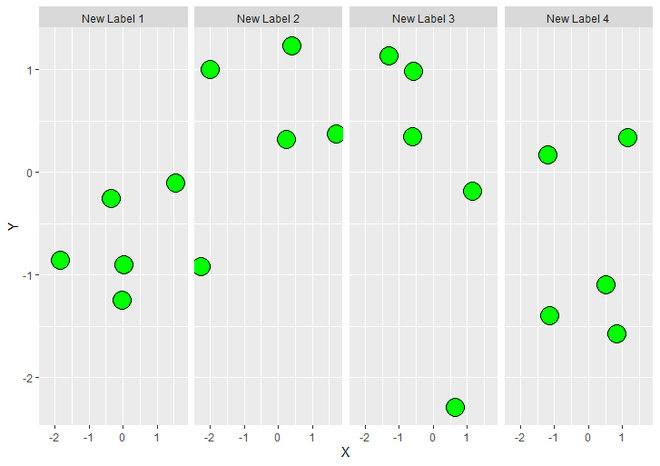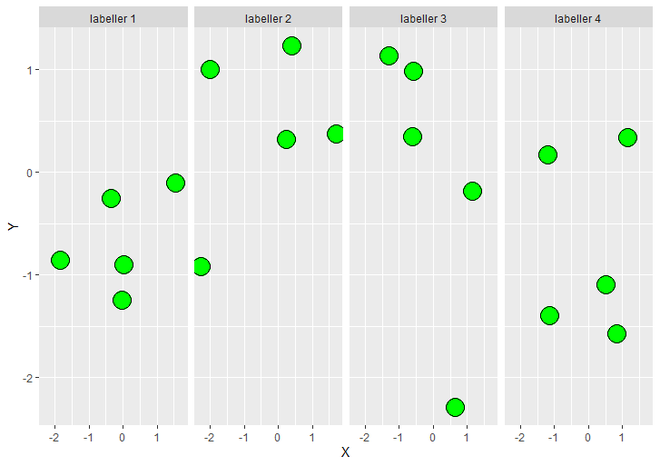Related Articles

# Change Labels of GGPLOT2 Facet Plot in R

• Last Updated : 30 Jun, 2021

In this article, we will see How To Change Labels of ggplot2 Facet Plot in R Programming language.

To create a ggplot2 plot, we have to load ggplot2 package. library() function is used for that. Then either create or load dataframe. Create a regular plot with facets. The labels are added by default.

Example:

## R

 `library``(``"ggplot2"``) ``   ` `DF <- ``data.frame``(X = ``rnorm``(20),                                   ``                 ``Y = ``rnorm``(20),``                 ``LBLs = ``c``(``"Label 1"``, ``"Label 2"``, ``                          ``"Label 3"``, ``"Label 4"``))`` ` `ggplot``(DF, ``aes``(X, Y)) +                                     ``  ``geom_point``(size = 7, fill = ``"green"``, color = ``"black"``, shape = 21) +``  ``facet_grid``(. ~ LBLs)`

Output :FacetPlot using ggplot2

Now let us explore different approaches to change the labels.

### Method 1: Combine Label Variable with Facet Labels

If we want to combine Label Variable (LBLs) to Facet Labels (Values of LBLs), then for that we simply have to use labeller parameter of facet_grid() and set label_both to its value.

Example 1:

## R

 `library``(``"ggplot2"``) ``   ` `DF <- ``data.frame``(X = ``rnorm``(20),                                   ``                 ``Y = ``rnorm``(20),``                 ``LBLs = ``c``(``"Label 1"``, ``"Label 2"``,``                          ``"Label 3"``, ``"Label 4"``))`` ` `ggplot``(DF, ``aes``(X, Y)) +                                     ``  ``geom_point``(size = 7, fill = ``"green"``, color = ``"black"``, shape = 21) +``  ``facet_grid``(. ~ LBLs, labeller = label_both)`

Output :Combine Label Variable with Facet Labels

### Method 2: Using levels()

As we discussed previously the LBLs variable of DataFrame is responsible for Labels of Facet, so now we will create a new DataFrame and assign old DataFrame DF to it. Then simply change the Values of Labels variable of the new DataFrame. Here we use levels() function to set the attribute of a variable in a new manner.

Syntax : levels(x) <- value

Parameter :

• x : A Data Object eg. Vector.
• Value : Named List specifying how to rename the levels.

Return : Set (Rename) the value of vector.

Example 2:

## R

 `library``(``"ggplot2"``) ``   ` `DF <- ``data.frame``(X = ``rnorm``(20),                                   ``                 ``Y = ``rnorm``(20),``                 ``LBLs = ``c``(``"Label 1"``, ``"Label 2"``, ``                          ``"Label 3"``, ``"Label 4"``))``   ` `newDF <- DF``levels``(newDF\$LBLs) <- ``c``(``"New Label 1"``, ``"New Label 2"``, ``                        ``"New Label 3"``, ``"New Label 4"``)`` ` `ggplot``(newDF, ``aes``(X, Y)) +             ``  ``geom_point``(size = 7, fill = ``"green"``, color = ``"black"``, shape = 21) +``  ``facet_grid``(. ~ ``levels``(LBLs))`

Output :Changed Facet Labels using levels()

### Method 3: Using labeller parameter

The labeller can be a function, or it can be a named character vector (i.e. parameter), which can take either value or variable. labeller() function, when assigned to labeller parameter of facet_grid(), takes named arguments of the form ‘variable = value’ and changes the labels manually.

Example 3:

## R

 `library``(``"ggplot2"``) ``   ` `DF <- ``data.frame``(X = ``rnorm``(20),                                   ``                 ``Y = ``rnorm``(20),``                 ``LBLs = ``c``(``"Label 1"``, ``"Label 2"``,``                          ``"Label 3"``, ``"Label 4"``))`` ` `new <- ``c``(``"labeller 1"``, ``"labeller 2"``,``         ``"labeller 3"``, ``"labeller 4"``)``names``(new) <- ``c``(``"Label 1"``, ``"Label 2"``, ``                ``"Label 3"``, ``"Label 4"``)`` ` `ggplot``(DF, ``aes``(X, Y)) +             ``  ``geom_point``(size = 7, fill = ``"green"``, color = ``"black"``, shape = 21) +``  ``facet_grid``(. ~ LBLs, labeller = ``labeller``(LBLs = new))`

Output:Changed Facet Labels using labeller

My Personal Notes arrow_drop_up